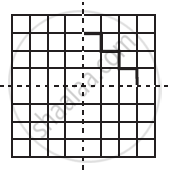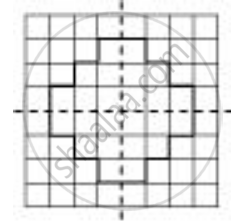Share

# Copy the Diagram and Complete the Given Shape to Be Symmetric About the Mirror Line(S): - 2 - CBSE Class 7 - Mathematics

ConceptLines of Symmetry for Regular Polygons

#### Question

Copy the diagram and complete the given shape to be symmetric about the mirror line(s):#### Solution

The given figure can be completed about the given mirror lines as followsIs there an error in this question or solution?

#### APPEARS IN

NCERT Solution for Mathematics for Class 7 (2018 to Current)
Chapter 14: Symmetry
Ex. 14.10 | Q: 6.2 | Page no. 270
Solution Copy the Diagram and Complete the Given Shape to Be Symmetric About the Mirror Line(S): - 2 Concept: Lines of Symmetry for Regular Polygons.
S